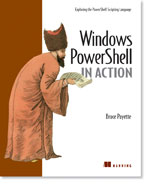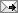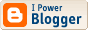# /\/\o\/\/ PowerShelled

This blog has moved to http://ThePowerShellGuy.com Greetings /\/\o\/\/\$AtomFeed = ("Atom.xml")
\$PreviousItems = (" Scripting Games almost over "," The Scripting Games Event 6 in MSH "," Scripting Games week 2 "," Monad Links "," MSH Concentration (scripting games part 4) "," scripting games part 3 "," 2006 Winter Scripting Games (part 2) "," Monad hosting "," Get current user in Monad "," Different ways to do things in Monad, and some links "," ")

## scripting games Last Day MSH answers for event 7 and 8

The Final day of the scripting games,

Anwers to Event 7 and Event 8 are posted on the Scripting Games Home. (where you can also find the needed files and original events)

And I will post the MSH versions I made as aswers here, lets start with event 7.

Event 7

In my Vbscript answer I used this way to translate the time,

Total = (TimeValue("00:" & time1) + TimeValue("00:" & time2))

for the rest my script was basicly the same.

with the same construction in MSH I came to this one-liner for the 10 points answer :

# 10 Points one-liner unsorted

gc C:\Scripts\Event_7.txt | foreach {\$l = \$_.split(",");"\$(\$l;(([datetime]`"00:\$(\$l)`").Add([timespan]`"00:\$(\$l)`")).tostring('m:ss'))"}

but to get the full 15 points you need to sort the list, how to do that ?
now does MSH have a sort (sort-object) but its not very usefull on this output.

the trick here is to use select-object , with select I came to the following onliner :

# using select to be able to sort to get the 15 points

gc C:\Scripts\Event_7.txt | select {\$_.split(",")},@{E={([datetime]"00:\$(\$_.split(','))").add([timespan]"00:\$(\$_.split(','))").tostring('m:ss')};N="t"} | sort -desc t | ft -h

(as no columns where asked, I just renamed the last to make the sort command not to long ;-))

but to get a nice list and because this is a bit cryptic, I also made this version,

# Write it out a litle bit more and rename the first column
# so we can show the headers

get-content C:\Scripts\Event_7.txt | select @{Expression={\$_.split(",")};Name="Competitor"},
@{Expression={
\$Time1 = ([datetime]"00:\$(\$_.split(','))")
\$Time2 = ([timespan]"00:\$(\$_.split(','))")
};Name="Time"} | sort -property Time -descending :\$(\$_.split(','))").add([timespan]"00:\$(\$_.split(','))").tostring('m:ss')};N="t"} | sort -desc t | ft -h

*Note* technicaly this is still a one-liner I just entered some linebreaks and spaces to format it a bit.

this will add meaningfull descriptions to the table generated.
the naming and formating af data in select is not yet in the Help but tor more information see also the good explanation in ::MSH:: from DontBotherMeWith Spam about the first MP3 entry on my blog where I use this also.

for another even more powerfull way to do this kind of things (and more) in MSH (with add-member) see the second post about MP's Some fun With Monads Add-Member, MP3 files and COM

But time to go on to the last event

Event 8

For this I also made 2 solutions,

The first one is basicly the same as the Vbscript version.

- It connects to access,
- It does transform the data in the SQL query
- It outputs the data.

# Open connection to the Access DataBase

\$mdb = "c:\scripts\event_8.mdb"
\$ConnString = "Provider=Microsoft.Jet.OLEDB.4.0;Data Source=\$mdb"
\$Conn = new-object System.Data.OleDb.OleDbConnection(\$connString)
\$conn.open()

# do processing in SQL Query

\$SQL = "SELECT MedalWinners.Country,"
\$SQL += " Sum(MedalWinners.Gold) AS Gold,"
\$SQL += " Sum(MedalWinners.Silver) AS Silver,"
\$SQL += " Sum(MedalWinners.Bronze) AS Bronze,"
\$SQL += " Sum([Gold]+[silver]+[bronze]) AS Total"
\$SQL += " FROM MedalWinners GROUP BY MedalWinners.Country"
\$SQL += " ORDER BY Sum([Gold]+[silver]+[bronze]) DESC;"

\$cmd = new-object System.Data.OleDb.OleDbCommand(\$SQL,\$Conn)
\$dt = new-object System.Data.dataTable
[void]\$da.fill(\$dt)

\$dt | ft -auto

You can see the formatting part is a bit easier (I used the spaces function in my VbScript answer), for the rest is basicly the same.
also I did the datebase connection already for Excel, see Using ADO to get data from Excel to Dataset in Monad. and SQL see Getting and Working With SQL Server data in Monad

And the SQL query I did just create in Access, only I changed the Query by removing the Sum that Access does append automatic so I do not have to make the headers myself as the scripting guys did ;-)

So to make it a bit more an event I made a second version that just gets the original table and does the processing of the data on the MSH side.

this second version looks like this :

# Alternative way, do the processing on MSH side

\$mdb = "c:\scripts\event_8.mdb"
\$ConnString = "Provider=Microsoft.Jet.OLEDB.4.0;Data Source=\$mdb"
\$Conn = new-object System.Data.OleDb.OleDbConnection(\$connString)
\$conn.open()

\$cmd = new-object System.Data.OleDb.OleDbCommand("select * from medalwinners",\$Conn)
\$dt = new-object System.Data.dataTable
\$da.fill(\$dt)

\$Total.expression = "(Gold+silver+bronze)"

\$dt | select -unique country | select country,
@{e={\$dt.compute("Sum(Bronze)","Country = '\$(\$_.country)'")};n='Bronze'},
@{e={\$dt.compute("Sum(Silver)","Country = '\$(\$_.country)'")};n='Silver'},
@{e={\$dt.compute("Sum(Gold)","Country = '\$(\$_.country)'")};n='Gold'},
@{e={\$dt.compute("Sum(Total)","Country = '\$(\$_.country)'")};n='Total'} |
sort Total -desc | ft -auto

You can see that it just append a column for the type Int, and adds a expression to it.
Then I do a select -unique to get only the countries
while I loop through them I use the Compute Method of the datatable, that takes a Expression and a filter as arguments, to sum the medals for every country , like in the first sample I did this all within a Select statement inside calculated properties.

and then as promised, as a bonus, the alternative ending for The Scripting Games Event 6 in MSH
as you can guess by now it uses SELECT ;-)

# Format and output results

\$words | select @{e={\$_};n='Word'},@{e={\$found -contains \$_};n='Found'} | sort found -desc | ft -auto

that was it for this final day, hoped you liked this series as I did doing the original Games and the MSH side-o-lypics series and thanks from here to the scripting guys for aranging the Scripting games, and hope there will be some MSH events in it next year.

Enjoy,

Greetings /\/\o\/\/

PS you can find the needed files and orignal events from the main pa
Tags :Anonymous
Thanks for these posts - they were a real joy.Anonymous
Yeah - these are pretty cool. Here's an idea for a tweak on the solutions. If you use multiple assignments and casts, you can make things even shorter and a bit more efficient by getting rid of the multiple splits. Here's the revised 10 pointer:

gc C:\Scripts\Event_7.txt | foreach{\$n,[Timespan]\$t1,[timespan]\$t2 = \$_.Split(','); "\$n \$(\$t1+\$t2)" }

And here's the sorted version. (Note the junk '1' being piped into select though.)

gc C:\Scripts\Event_7.txt | foreach{\$n,[Timespan]\$t1,[timespan]\$t2 = \$_.Split(','); 1 | select @{n="name";e={\$n}},@{n="span";e={\$t1+\$t2}}} | sort span | ft -h

-bruce/\/\o\/\/

@bruce,

there are 2 gotha's in the script/event that you missed in your solution,

1) times are in Minute and seconds,
hence I add "00:" before I translate to datetime,

2) the first cast I do to DateTime, as TimeSpan does not have the toString overload needed to put back the output in Minutes and seconds (as asked).

Your solution will "translate" the times from minutes in hours.
(and you have no room to put the "00:" in front)

gr /\/\o\/\/Anonymous
gc C:\\Scripts\\Event_7.txt | foreach{\$n,[string]\$t1,[string]\$t2=\$_.split(\',\') ;\$t1 | foreach{[int]\$m1,[int]\$s1=\$_.spli(\':\')}; \$t2 | foreach {[int]\$m2,[int]\$s2=\$_.split(\':\')};\$temp=[datetime]\"00:00:00\";\$temp=\$temp.addminutes(\$m1+\$m2);\$temp=\$temp.a
ddseconds(\$s1+\$s2);\"\$n \$(\$temp.tostring(\'m:ss\'))\"}
##########################
A kind of combination.But it\'s so long.I just afraid to publish the script for shame.applepwc
Sorry for double post.In the previous post,because I have using IE proxy server.So the text transmitting generate some errors.
##############################
gc C:\Scripts\Event_7.txt | foreach{\$n,[string]\$t1,[string]\$t2=\$_.split(',') ;\$t1 | foreach {[int]\$m1,[int]\$s1=\$_.split(':')}; \$t2 | foreach {[int]\$m2,[int]\$s2=\$_.split(':')};\$temp=[datetime]"00:00:00";\$temp=\$temp.addminutes(\$m1+\$m2);\$temp=\$temp.a
ddseconds(\$s1+\$s2);"\$n\$(\$temp.tostring('m:ss'))"}/\/\o\/\/
@applepcw

A good alternative to translate the date,
only you can shorten it up by removing the Cast and the Foreach, as they are unneeded, and I did put the minutes and seconds on one line.

see examples below

gr /\/\o\/\/

# original over more lines

gc C:\Scripts\Event_7.txt | foreach{
\$name,\$t1,\$t2 = \$_.split(',')
\$t1 | foreach {[int]\$m1,[int]\$s1 = \$_.split(':')}
\$t2 | foreach {[int]\$m2,[int]\$s2 = \$_.split(':')}
"\$name \$(\$time.tostring('m:ss'))"
}

# change in time cast

gc C:\Scripts\Event_7.txt | foreach{
\$name,\$t1,\$t2 = \$_.split(',')
\$m1,\$s1 = \$t1.split(':')
\$m2,\$s2 = \$t2.split(':')
"\$name \$(\$time.tostring('m:ss'))"
}/\/\o\/\/
Shortened the time to 0 and put on one line again :

gr /\/\o\/\//\/\o\/\/
gc C:\Scripts\Event_7.txt | foreach{\$n,\$t1,\$t2 = \$_.split(',');\$m1,\$s1 = \$t1.split(':');\$m2,\$s2 = \$t2.split(':');"\$n \$(([datetime]0).addseconds(((\$m1+\$m2)*60)+\$s1+\$s2).tostring('m:ss'))"}/\/\o\/\/
just for the fun of it :

gc C:\Scripts\Event_7.txt | foreach{\$n,\$t1,\$t2 = \$_.split(',');\$m1,\$s1 = \$t1.split(':');\$m2,\$s2 = \$t2.split(':');\$s=((\$m1+\$m2)*60+\$s1+\$s2);"\$n \$([int](\$s/60)):\$((\$s%60).tostring('00'))"}Anonymous
@/\/\o\/\/
May be some cast is needed.

Try your original script as below,the consequence isn't right.

###########################################
The reason is the \$m1,\$m2,\$s1,\$s2 are all "string" type./\/\o\/\/
@applepcw

gr /\/\o\/\/

<< Home

## Archives

October 2005   November 2005   December 2005   January 2006   February 2006   March 2006   April 2006   May 2006   June 2006   July 2006   August 2006   September 2006   October 2006   November 2006   December 2006

\$Links = ("PowerShell RC1 Docs"," PowerShell RC1 X86"," PowerShell RC1 X64"," Monad GettingStarted guide"," Monad Progamming Guide"," Monad SDK"," Monad videos on Channel 9"," MSH Community Workspace"," scripts.readify.net "," MonadSource"," www.reskit.net"," PowerShell Blog"," Under The Stairs"," computerperformance powershell Home"," proudlyserving"," MSH on wikipedia"," MSHWiki Channel 9"," Keith Hill's Blog"," Precision Computing"," PowerShell for fun"," MSH Memo (Japanese)"," monadblog")